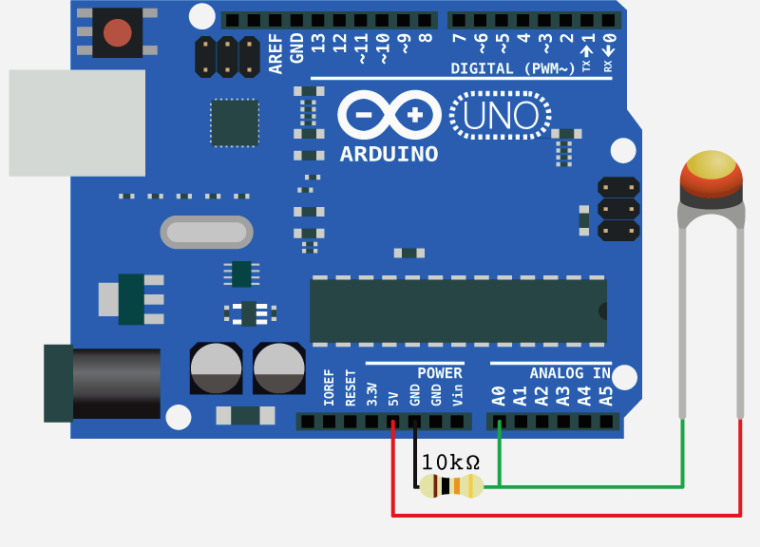NTC temperature sensor

• @Shaun said in NTC temperature sensor:

apin=adc.channel(attn=3, pin='P16') # should work for you

Great, this changes the bevahiour and looks much better now. Where do I find documentation about this? The official documentation about ADC doesn't talk f.e. about the attn parameter.

• @tobru The problem is that the ADC is saturating.
Try change the ADC input attenuation, which will increase the ADC full scale conversion voltage.
attn = (0 = 0db : 1.1V max), (1 = 2.5db : 1.4V max), (2 = 6db : 1.9V max), (3 = 11db : 3.2V max)
apin=adc.channel(attn=3, pin='P16') # should work for you

Edit:
Be warned that the ESP32 ADC is nonlinear and rather noisy.
To reduce the jitter in readings, I take 5 readings at 100us cadence, discard the highest and lowest and average the remaining 3.

The non linearity has to be dealt with using a lookup table or conversion function for now.

• "The 10k resitor" should be the same value as your termistor resistance (it can be different than 10k, I used the one with 50k resistance). This schematic worked for me with esp8266 (on LUA), so I don't think it have a problem with 3.3V

• Ok, looks like the 10K resistor doesn't fit here with 3V3. Nevertheless I'm a bit lost and not sure how to chose the correct resistor and calculate the temperature from the measured value.

• Thanks a lot for the explanation. But I don't get it yet =(

I connected 3V3 to the thermistor on one end, G3 (P16) and the 10k resistor to the other end of the termistor. GND is connected to the 10k resistor (other end).

10K Thermistor

+-----------+          +-------+
|           |          |       |
|       3V3 +----------+       +----+
|           |          +-------+    |
|           |                       |
|       G3  +-----------------------+
|           |                       |
|           |          +-------+    |
|           |          |       |    |
|       GND +----------+       +----+
+-----------+          +-------+

10K Resistor

All I get is the value 4095 when I read it with the following code:

import machine
import time

for i in range(10):
val = apin()
print(val)
time.sleep(1)

What did I understand wrong?

• @tobru said in NTC temperature sensor:

10K NTC

this is simple analog sensor - thermistor
connect it to any analog pin - here you have sample for arduino
but sample is valid for lopy - only difference is that you connect it to 3V3 pin not to 5V pin because pycom board are 3V3 compatibile and not tollerant to 5V

![alt text](image url)

here you have pinout of lopy